Back to Nautical Displacement Buoyancy FWA Movement of Centre of Gravity Angle of Loll Curves of Stat. Stability List Free Surface Effetcs Trim

Stability

List

Definitions

Roll: The action of a vessel involving a recurrent motion, usually caused by wave action.

Heel: Semi-permanent angle of inclination caused by external forces, such as high-speed turns, beam winds, and seas.

List: Permanent angle of inclination, caused by:

1. Ship’s Center of Gravity transversely shifted from centerline.

2. Negative Metacentric Height (-GM)

3. Combination of Gravity off-centerline and –GM

Moment To Heel 1o Equation

When a ship experiences an Inclining Moment (IM) the vessel will list or heel until the Righting Moment (RM) is equal to the Inclining Moment (RM = IM). The Inclining Moment is simply a force acting through some distance.

IM = w x d

This is only true when the ship has a negligible heel or list.As the vessel inclines, the distance between the forces changes.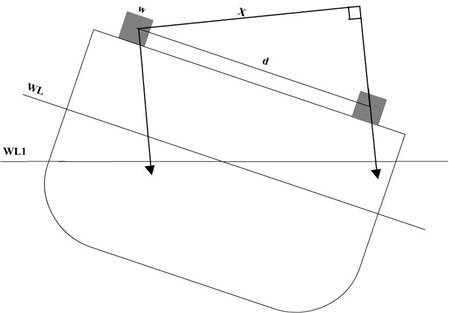A relationship can be developed to solve for the distance between forces for all angles of heel. Using an expanded drawing of the triangle from the above diagram: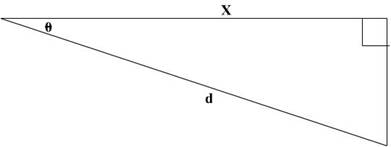Using the cosine equation to solve for the distance X:

X = d x cos θ

Therefore:

IM = w x d x cos θ

A Righting Moment is created by the ship to keep itself upright. In this case, the force is equal to the ship’s displacement (WF) and the distance is the ship’s righting arm (GZ) at each particular angle of heel.

RM = WF x GZ

The Righting Arm (GZ) changes with inclination of the ship. Using the relationship derived for small angles of heel:

GZ = GM x sin θ

NOTE: This relationship holds true for angles less than 7°-10°

Therefore:

RM = GM x WF x sin θ

The initial premise was that RM = IM:

W x d x cos θ = GM x WF x sin θ

Transferring cosine θ to the right:

(sin θ / cos θ) = tan θ

w x d = GM x WF x tan θ

Choosing a specific angle, the moment (w x d) required to create that list or heel can be found. Using 1o:

tan 1o = 0.01746

Therefore:

MH 1˚ = GM x WF x 0.01746

This formula is valid for angles less than 10o due to movement of the metacenter. To check this formula for all inclinations less than 10o, a comparison between the MH10o and 10 times MH1o is made.

MH 10˚ = GM x WF x tan 10˚ -vs-      10 x (MH 1˚ = GM x WF x 0.01746)

MH 10˚ = GM x WF x (0.01746)

And

10 x (MH 1˚) = 10 x GM x WF x (0.01746)

There is a 0.0017 difference over the 10˚range. This error is negligible. The list equation can now be used.

LIST = (w x d) / MH 1˚

Example

Your ship has a 1.5o list to starboard. There are 50 MT of cargo placed on the starboard side. The stevedores want to know how far to transfer the cargo to correct the list.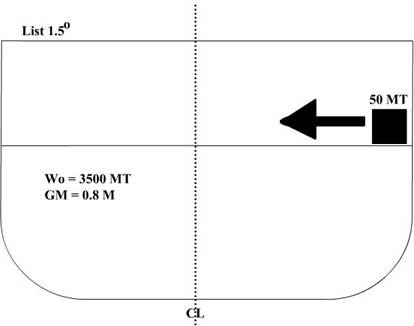Step 1: Calculate MH1o:

MH 1˚ = GM x WF x (0.01746)

MH 1˚ = 0.8 M x 3500 MT x (0.01746)

MH 1˚ = 48.8 M

Step 2: Use the list equation to solve for distance:

List = (w x d) / MH 1˚

Or, 1.5˚ = (50 MT x d) / 48.8 M

d = (1.5 x 48.8) / 50 = 1.464 M

Example

Your ship has a 2° list to port. The CO wants it corrected. There are 15 cbm of fuel in the port wing tank (sp.gr. 0.94). The starboard wing tank is empty. Correct the list using the fuel and a set of 5 cargo pallets (8 MT each). The cargo pallets may only be moved 5 M to starboard before hitting the bulkhead. How long will it take to correct the list? Pump capacity is 40 cbm per hour.

WO = 12500 MT

KM = 7.1 M

KG = 6.02 M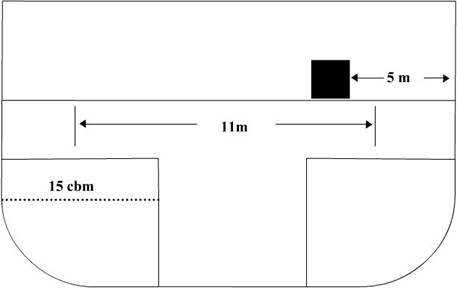Step 1: Calculate MH1°:

MH 1˚ = GM x WF x (0.01746)

MH 1˚ = (7.1 – 6.02) x 12500 x (0.01746)

MH 1˚ = 235.7

Step 2: Calculate the amount of list corrected by shifting fuel:

Weight of fuel = 15 x 0.94 = 14.1 MT

List = (w x d) / MH 1˚

Or,

List = (14.1 x 11) / 235.7 = 0.66˚

Step 3: So far, we have corrected 0.66o of the 2o list. Using the pallets, we will correct for the remaining 1.34o list.

List = (w x d) / MH 1˚ or

d = (1.34 x 235.7) / 40 = 7.9 M

Step 4: Finally, calculate how long it takes to transfer 15 cbm of fuel when the pump capacity is 40cbm/ hour.

Time = (15 cbm / 40 cbm/h) = 0.375 hr x 60 = 22.5 minutes

Assuming it takes less than 22.5 minutes to move the 5 pallets, this is the time required to correct the list.

Important:

1. When attempting problems on List, first find out the GM of the vessel (if the KG has to be calculated then do so) if it has not been stated.
2. If there are more than one shifting/ loading/ discharging involved then tabulate the moments and get the final moment (w x d) to either port or to starboard.

Example:

A ship of 8000 tonnes displacement has KM = 8.7 m, and KG = 7.6 m. The following weights are then loaded and discharged:

1. Load 250 tonnes cargo KG 6.1m and centre of gravity 7.6m, to starboard of the centre line.
2. Load 300 tonnes fuel oil KG 0.6m, and centre of gravity 6.1m, to port of the centre line.
3. Discharge 50 tonnes of ballast KG 1.2m, and centre of gravity 4.6m, to port of the centre line.

Find the final list.

 Weight KG Moment about Keel (V-M) Orig. Disp. 8000 7.6 60800 Load 250 6.1 1525 Load 300 0.6 180 Total 8550 62505 Disch. -50 1.2 -60 Final Disp. 8500 62445

Final KG = Final Moment / Final displacement        = 62445 / 8500

KG                        =          7.34

KM            =          8.7

Therefore, GM       =          1.36

 w d Listing moment Port Stbd 250 7.6 1900 50 4.6 230 300 6.1 1830

From above we have Port: 1830 and Stbd: 2130

Therefore the final listing moment (w x d) = 300 to stbd.

Now, MH 1˚ = GM x WF x 0.01746         = 1.36 x 8500 x 0.01746         = 201.8376

List = (w x d) / MH 1˚        = 300 / 201.8376         = 1.49˚ to stbd.
Inclining Experiment

The inclining experiment is completed upon commissioning of the vessel.

It is performed to obtain accurately the vertical height of the ship’s center of gravity above the keel (KG).

Procedures:

The shipyard at which the inclining experiment is to be performed will issue a memorandum to the ship outlining the necessary work to be done by ship’s force and by the yard to prepare the ship for inclining.

1. Liquid load will be in accordance with the memorandum.

2. Inventory of all consumables to be made by ship’s crew and inclining party.

3. Inclining weights are placed on centerline.

4. Freeboard is measured, and a photo of the drafts is taken.

5. Salinity of saltwater is measured.

8. Pendulums set up forward, midships, and aft.

9. Weights are moved off-centerline.

10.Inclination of the ship measured.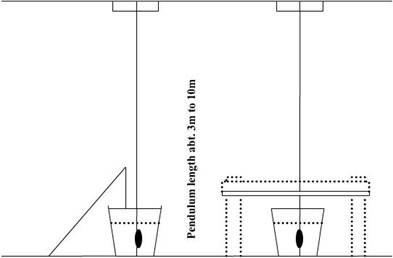Measurements are taken for several weight movements both port and starboard. The Naval Architect then uses the following equation:Where:

w = Inclining Weights (LT)

d = Athwartships Distance Weights Were Moved (FT)

WF = Displacement of Ship (LT, with Inclining Weights)

tan θ = Movement of PendulumLength of Pendulum

The inclining experiment measures GM accurately, and since the ship’s drafts are known, KM can be found, KG is then found using KG = KM - GM.